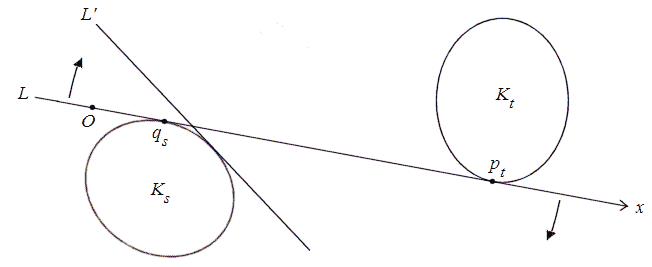# A ley-hunting lemma

The typescript of these notes is dated 28 Nov 1985. The lemma may be useful in computer simulations of ley-hunting. MB, May 2013

Let K1,,Kr be bounded closed convex plane sets, none of which is contained in the intersection of the rest. Suppose there exists a straight line L such that

 L∩Ki≠∅ (1≤i≤r).
(1)

Then we may assume that L is an external tangent to two of the Ki.

Proof  Suppose not. Regard L as a movable line. In L, fix an origin O and a direction Ox. Let the words “left” and “right” refer to an observer looking along Ox. Let LKi when non-empty be the interval pixqi. We show that for any positive integer s there exist a labelling of the Ki and a position of L such that

 L is tangent to Ks;
(2)
 Ki∩Ki+1=∅ (1≤i≤s−1);
(3)
 qi
(4)
Fig. 1First move L to the left without rotation until (1) fails. In the limiting position L is tangent to some Kt. Label so that t=1; then (2) to (4) hold with s=1.

Suppose (2) to (4) hold for some s. Let K lie to the right (resp. left) of L. Roll L clockwise (resp. anticlockwise) round the boundary of Ks and let θ be the resulting azimuth of Ox.

If (1) holds for all θ then Ks is contained in every other Ki, contrary to hypothesis; hence (1) eventually fails. In the limiting position L is tangent to some Kt with ts. By hypothesis [i.e. since the result is assumed false] Kt must lie to the left (resp. right) of L. Hence L can be rolled through a further small angle to a position L that separates Ks and Kt with LKt=. Hence KsKt=0 and qs<pt.

As L moves to the limiting position, the pi and qi vary continuously with θ, and by (3) qipi+1 throughout. Hence at the limiting position (4) holds, so that pi<pt for 1is. Hence we can label so that t=s+1. This completes the inductive step.

Hence for all s there exist s distinct Ki: contradiction.

Remark  If there are infinitely many Ki the result need not hold; e.g. let Ki be the line segment joining (i,±1/i).